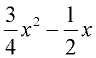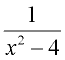Polynomial Basics Terms of Use    Contact Person: Donna RobertsDirections: Answer these questions pertaining to polynomials. Check each answer when finished.

1.
What is the degree of the polynomial:
5x3 + 4x2 - 6x +7

Choose:
 5 2 3 42.
What is the degree of the polynomial:
-8a4 + 4a5 - 9a + 12

Choose:
 5 2 3 43.
Which choice is not a polynomial?

Choose:
 x3+ 3x2 - 6x + 1 x2+ x-1 x9y14 + 30 64.
Which choice is a polynomial?

Choose: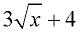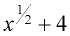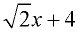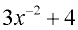5.
The set of polynomials is closed under addition, subtraction, multiplication and division.

Choose:
 True False6.
Which word describes the polynomial:
3x2 + 4x + 2
?

Choose:
 monomial binomial trinomial linear7.
The exponents used in polynomials belongs to which of the following sets?

Choose:
 {1, 2, 3, 4, ... } {0, 1, 2, 3, 4, ... } {2, 3, 4, 5, ... } {..., -1, 0, 1, 2, 3, ...}8.
In the polynomial 4x - 3x2 + 5, which choice is the "leading coefficient" ?

Choose:
 -3 5 3 49.
In the polynomial 4x - 3x2 + 5, which choice is the "constant term" ?

Choose:
 -3 5 -3x2 4x10.
Which choice is NOT a polynomial ?

Choose:
 -21x3 - 100 0.2x2 (0.3x1 + 0.1)